# Numbers

By how many is the difference of numbers 8 and 34 less than its product?

Correct result:

x =  246

#### Solution: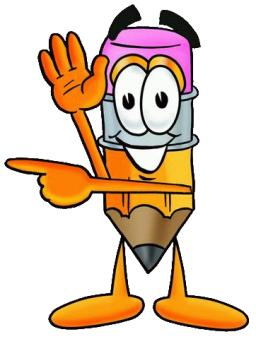We would be pleased if you find an error in the word problem, spelling mistakes, or inaccuracies and send it to us. Thank you!## Next similar math problems:

• How manzBy how many is the product of the numbers 328 and 7 greater than its sum?
• Grower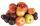Grower harvested 190 kg of apples. Pears harvested 10 times less. a) How many kg pears harvested? b) How many apples and pears harvested? c) How many kg harvested pears less than apples?
• Grandma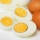Grandma bought two 10 pack of eggs. She used 8 eggs on the cake, 2 times less on the omelette and 4 more eggs for breakfast. How many eggs did it stand for?
• Algebra problemThis is algebra. Let n represent an unknown number. 1. Eight more than the number n 2. Three times the number n 3. The product of the number n and eight 4. Three less than the number n 5. Three decreased by the number n
• Beautiful alleyIn the alley 4 rows of 10 trees and 5 rows of 8 trees blossomed. Six of the trees have already blossomed. How many trees still bloom in the alley?
• Product of the sum and differenceCalculate the product of the sum and difference of numbers -7 and -2.
• Operations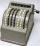Sum of the numbers 1.01 and 3.35 multiply by the difference of numbers 6.69 and 1.39.
• CDs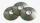Adam has 6 CDs more than Filip and 7 CDs less than Dominica. Filip has 6 CDs. How many CDs has Adam and how many CDs has Dominika?
• Integers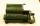May be the sum of two integers less than their difference?
• SubtractionTest what do you know about the subtraction of two numbers: a) make a difference if the minuend 4,307,288 and subtrahend is 472008 b) minuend is 4000 more than subtrahend. What's the difference? c) the difference is 38900 less than the minuend. What is th
• Apples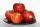Hanka has 6 apples more than Juro and 7 apples less than Mirka. Mirka has 23 apples. How many apples has Hanka and how many Juro?
• On the tree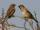15 squids and sparrows sat on the tree. Sparrows were 8 less than squids. How many sparrows were sitting at the tree?
• In the bowlThere are 12 pears in the bowl. Erik ate a third. How many pears remained in the bowl?
• Rabbits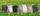In the hutch are 48 mottled rabbits. Brown are 23 less than mottled and white are 8-times less than mottled. How many rabbits are in the hutch?
• Boys and girls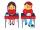In the class are 20 boys and 5-times less girls. How many girls are in the classroom? How many all children are in the class?How much and how many times is 72.1 greater than 0.00721?What is the product of 26 and 5? Write the answer in Arabic numeral. Add up the digits. How many of this is in a dozen? Divide #114 by this